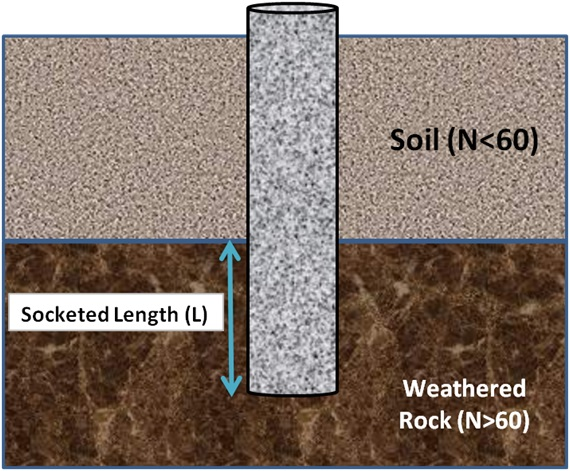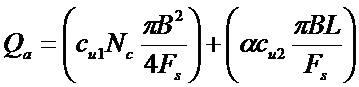# HOW TO CALCULATE PILE LOAD CAPACITY IN WEATHERED ROCK OR SOFT ROCK?Pile embeded in weathered rock

## Pile Load Capacity Calculation in Weathered or Soft Rock

This method of calculating pile load capacity was suggested by Cole & Stroud. Cole & Stroud used ‘N’ values or results of standard penetration tests to find allowable load capacity of the pile in weathered rocks.

The formula suggested by Cole & Stroud is given below. In the formula, the 1st term gives the end bearing resistance and the 2nd term gives the socketed bond strength between rock & pile.Where,

Qa = Allowable load capacity of pile, in kN

Cu1 = Shear strength of rock below the base of the pile, in kN/m2. (This is found out from fig-1, given below)

Cu2 = Average shear strength of rock in the socketed length of pile, in kN/m2. (This is found out from fig-1, given below)

Nc = Bearing capacity factor taken as 9

Fs = Factor of safety usually taken as 3

α = Adhesion factor, (recommended value is 0.9)

B = Minimum width of pile shaft (or diameter in case of circular pile) in m.

L = Socketed length of pile, in mFig-1-(Relation between shear strength of rock and SPT (N) values)

#### Notes to Remember

• The rock is considered to be weathered rock or soft rock if SPT values or N values are more 60. If less then it is considered as soil.
• If N values are more than 200, then, pile load capacity should be calculated on the basis of shear strength or rock.

### Reference

IS: 2911-Part-1-Sec-2

2 Comments
1.2.